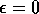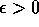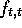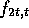Next: Conclusion Up: Byzantine Failures in Previous: Eventual Byzantine Agreement

## Approximate Agreement

The topic of approximate agreement is not strictly a problem of consensus, but is related and worth mentioning. We can modify the Byzantine Generals Problem so that each process inputs a real value rather than a binary value, and all processes must eventually decide on real values which are withinof each other. Notice that ifthen it is equivalent to the consensus problem with real values.

An algorithm described by Dolev et al.  works by successive approximation. That is, every round gets closer to the goal with a guarenteed convergence rate. The most interesting part of this algorithm is that it works both in synchronous and asynchronous systems (with). The conditions are similar to that of the consensus problem: all correct processes eventually halt with output values withinof each other, and all decided values are within the range of input values of all correct processes. For synchronous systems, the convergence rate depends on the ratio between the number of faulty processes and the total number of processes. Convergence is guarenteed when n > 3t. For asynchronous systems, convergence is guarenteed when n > 5t.

The synchronous algorithm basically works as follows. Each process sends its value to every other process. If a faulty process does not send a value, then some default value, say 0, is chosen. Each process then runs the functionon the n real values. Roughly speaking, the function f is chosen so as to eliminate the lowest and highest t values from the list and take the average of the rest; the faulty processes are thus unable to affect the convergence of the values. To show that it is necessary to have n > 3t, assume n=3t. If all t faulty processes send a very high value to correct process p and a very low value to correct process q, then p and q will be taking their averages on two completely different sets of numbers, and they will not converge. However, if n=3t+1, then p and q will have one overlapping value at their median, and will (very slowly) converge towards each other.

The asynchronous algorithm is the same as above, except that each process only waits for n-t messages, does not choose a default value, and runs the functionon the list. It is interesting to note that although exact consensus in a totally asynchronous system is impossible,can be chosen to be arbitrarily small to get as close to consensus as desired.Next: Conclusion Up: Byzantine Failures in Previous: Eventual Byzantine Agreement

Lawrence Kesteloot
Fri Jan 20 16:38:32 EST 1995